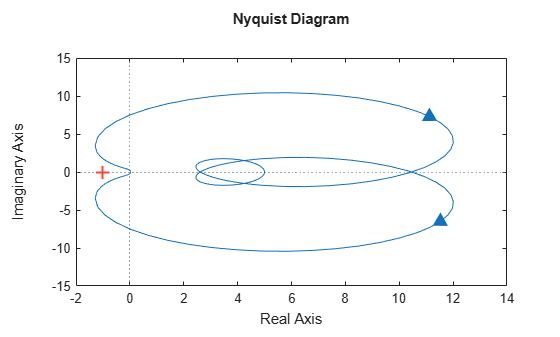# Series Interconnection of Passive Systems

This example illustrates the properties of a series interconnection of passive systems.

### Series Interconnection of Passive Systems

Consider an interconnection of two subsystems ${G}_{1}$ and ${G}_{2}$ in series. The interconnected system $H$ is given by the mapping from input $u$ to output ${y}_{2}$.In contrast with parallel and feedback interconnections, passivity of the subsystems ${G}_{1}$ and ${G}_{2}$ does not guarantee passivity for the interconnected system $H$. Take for example

`${G}_{1}\left(s\right)=\frac{5{s}^{2}+3s+1}{{s}^{2}+2s+1},\phantom{\rule{0.2777777777777778em}{0ex}}\phantom{\rule{0.2777777777777778em}{0ex}}\phantom{\rule{0.2777777777777778em}{0ex}}{G}_{2}\left(s\right)=\frac{{s}^{2}+s+5s+0.1}{{s}^{3}+2{s}^{2}+3s+4}.$`

Both systems are passive as confirmed by

```G1 = tf([5 3 1],[1,2,1]); isPassive(G1)```
```ans = logical 1 ```
```G2 = tf([1,1,5,.1],[1,2,3,4]); isPassive(G2)```
```ans = logical 1 ```

However the series interconnection of ${G}_{1}$ and ${G}_{2}$ is not passive:

```H = G2*G1; isPassive(H)```
```ans = logical 0 ```

This is confirmed by verifying that the Nyquist plot of ${G}_{2}{G}_{1}$ is not positive real.

`nyquist(H)`### Passivity Indices for Series Interconnection

While the series interconnection of passive systems is not passive in general, there is a relationship between the passivity indices of ${G}_{1}$ and ${G}_{2}$ and the passivity indices of $H={G}_{2}{G}_{1}$. Let ${\nu }_{1}$ and ${\nu }_{2}$ denote the input passivity indices for ${G}_{1}$ and ${G}_{2}$, and let ${\rho }_{1}$ and ${\rho }_{2}$ denote the output passivity indices. If all these indices are positive, then the input passivity index $\nu$ and the output passivity index $\rho$ for the series interconnection $H$ satisfy

`$\nu \ge -\frac{0.125}{{\rho }_{1}{\rho }_{2}},\phantom{\rule{0.2777777777777778em}{0ex}}\phantom{\rule{0.2777777777777778em}{0ex}}\phantom{\rule{0.2777777777777778em}{0ex}}\rho \ge -\frac{0.125}{{\nu }_{1}{\nu }_{2}}.$`

In other words, the shortage of passivity at the inputs or outputs of $H$ is no worse than the right-hand-side expressions. For details, see the paper by Arcak, M. and Sontag, E.D., "Diagonal stability of a class of cyclic systems and its connection with the secant criterion," Automatica, Vol 42, No. 9, 2006, pp. 1531-1537. Verify these lower bounds for the example above.

```% Output passivity index for G1 rho1 = getPassiveIndex(G1,'output'); % Output passivity index for G2 rho2 = getPassiveIndex(G2,'output'); % Input passivity index for H=G2*G1 nu = getPassiveIndex(H,'input')```
```nu = -1.2886 ```
```% Lower bound -0.125/(rho1*rho2)```
```ans = -2.4194 ```

Similarly, verify the lower bound for the output passivity index of $H$.

```% Input passivity index for G1 nu1 = getPassiveIndex(G1,'input'); % Input passivity index for G2 nu2 = getPassiveIndex(G2,'input'); % Output passivity index for H=G2*G1 rho = getPassiveIndex(H,'output')```
```rho = -0.6966 ```
```% Lower bound -0.125/(nu1*nu2)```
```ans = -6.0000 ```